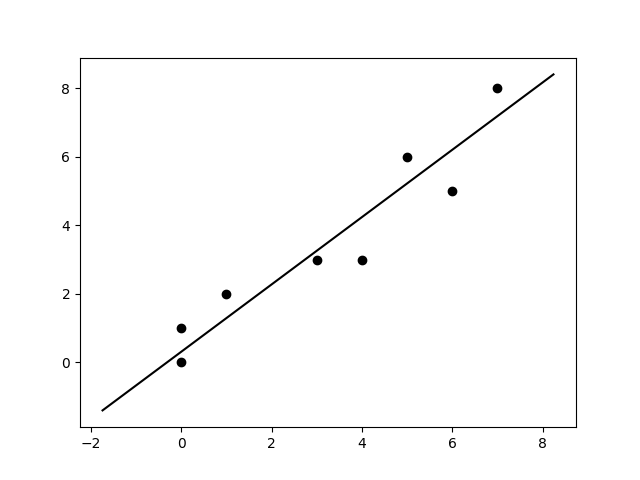# 2D Line of Best Fit¶

Fit a line to multiple 2D points.Out:

```(<Figure size 640x480 with 1 Axes>, <AxesSubplot:>)
```

```from skspatial.objects import Line, Points
from skspatial.plotting import plot_2d

points = Points(
[
[0, 0],
[0, 1],
[1, 2],
[3, 3],
[4, 3],
[6, 5],
[5, 6],
[7, 8],
],
)

line_fit = Line.best_fit(points)

plot_2d(
line_fit.plotter(t_1=-7, t_2=7, c='k'),
points.plotter(c='k'),
)
```

Total running time of the script: ( 0 minutes 0.102 seconds)

Gallery generated by Sphinx-Gallery